# Index Determination in DAEs using the Library indexdet and the ADOL-C Package for Algorithmic Differentiation

Prof. Dr. Computer Science (Artificial Intelligence, Software Engineering), Co-Founder AGISI.org at Computer Science Dept., Berlin School of Economics and Law
Aug. 5, 2012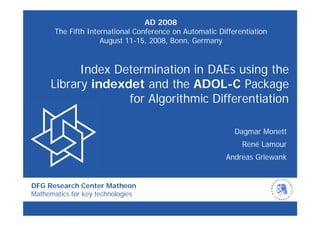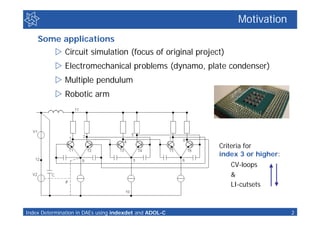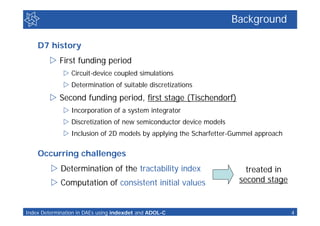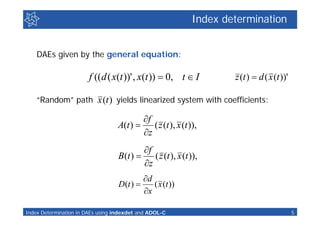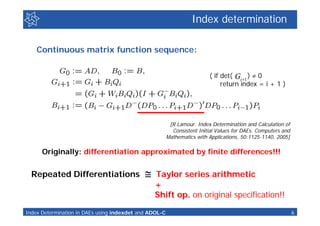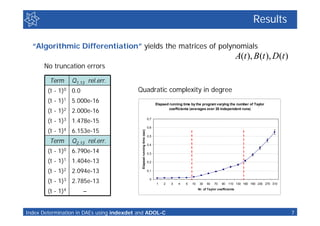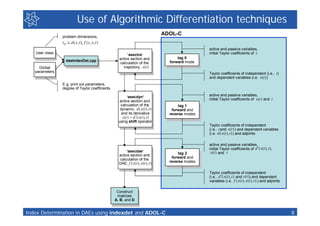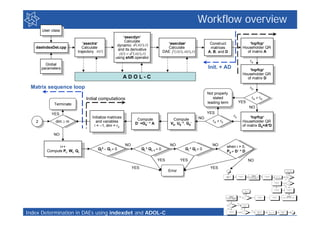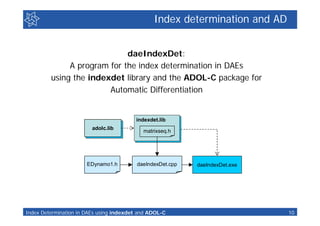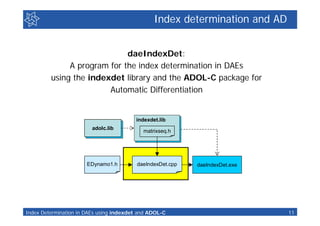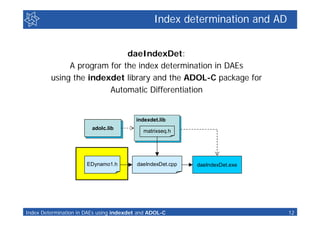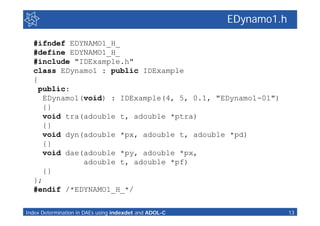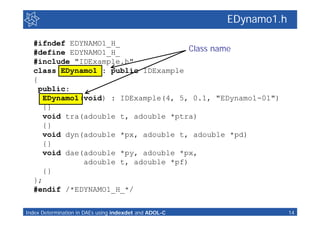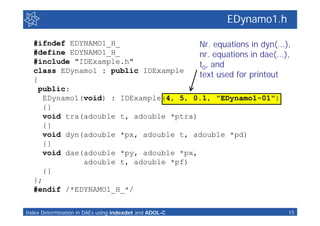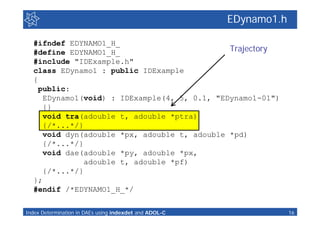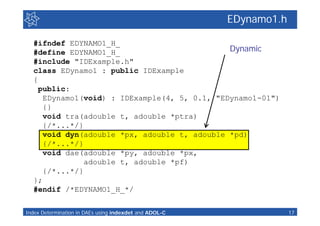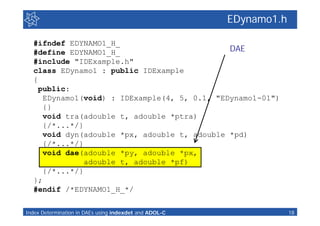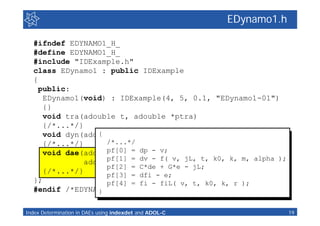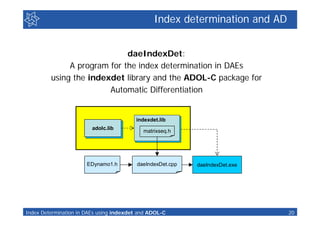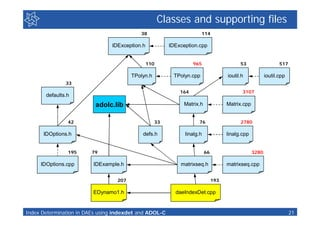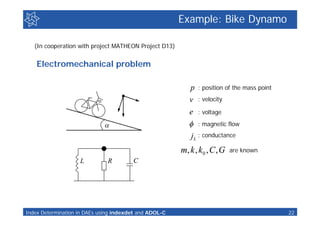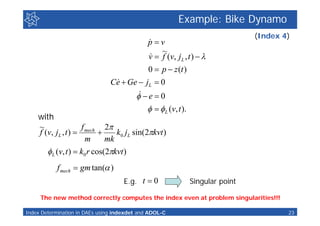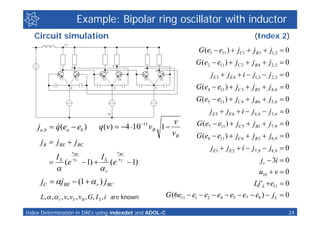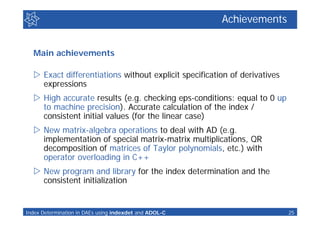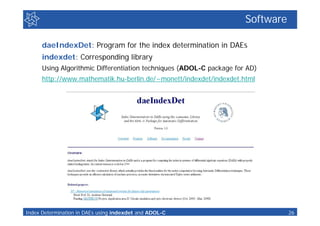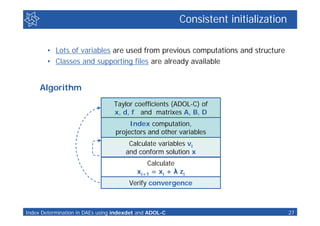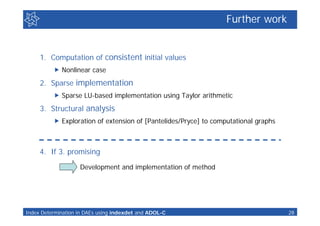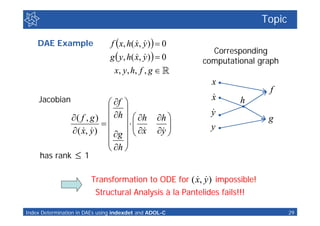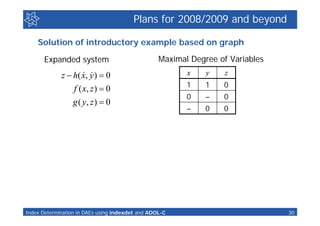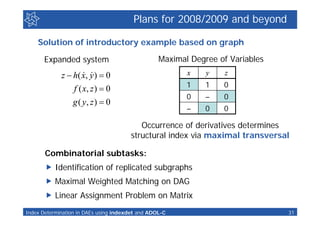1 of 31

### Index Determination in DAEs using the Library indexdet and the ADOL-C Package for Algorithmic Differentiation

1. AD 2008 The Fifth International Conference on Automatic Differentiation August 11-15, 2008, Bonn, Germany Index Determination in DAEs using the Library indexdet and the ADOL-C Package for Algorithmic Differentiation Dagmar Monett René Lamour Andreas Griewank DFG Research Center Matheon Mathematics for key technologies
2. Motivation Some applications  Circuit simulation (focus of original project)  Electromechanical problems (dynamo, plate condenser)  Multiple pendulum  Robotic arm Criteria for index 3 or higher: CV-loops & LI-cutsets Index Determination in DAEs using indexdet and ADOL-C 2
3. Background Project D7 Numerical simulation of integrated circuits for future chip generations • MATHEON - Mathematics for key technologies: Modeling, simulation and optimization of real-world processes • MATHEON is a Research Centre funded by the DFG, Deutsche Forschung Gemeinschaft (German Research Foundation) Index Determination in DAEs using indexdet and ADOL-C 3
4. Background D7 history  First funding period  Circuit-device coupled simulations  Determination of suitable discretizations  Second funding period, first stage (Tischendorf)  Incorporation of a system integrator  Discretization of new semiconductor device models  Inclusion of 2D models by applying the Scharfetter-Gummel approach Occurring challenges  Determination of the tractability index treated in  Computation of consistent initial values second stage Index Determination in DAEs using indexdet and ADOL-C 4
5. Index determination DAEs given by the general equation: f ((d ( x(t ))' , x(t ))  0, t  I z (t )  d ( x (t ))' “Random” path x (t ) yields linearized system with coefficients: f A(t )  ( z (t ), x (t )), z f B (t )  ( z (t ), x (t )), z d D(t )  ( x (t )) x Index Determination in DAEs using indexdet and ADOL-C 5
6. Index determination Continuous matrix function sequence: ( if det( Gi 1 )  0 return index = i + 1 ) [R.Lamour. Index Determination and Calculation of Consistent Initial Values for DAEs. Computers and Mathematics with Applications, 50:1125-1140, 2005] Originally: differentiation approximated by finite differences!!! Repeated Differentiations  Taylor series arithmetic + Shift op. on original specification!! Index Determination in DAEs using indexdet and ADOL-C 6
7. Results “Algorithmic Differentiation” yields the matrices of polynomials A(t ), B (t ), D(t ) No truncation errors Term Q1,13 rel.err. (t - 1)0 0.0 Quadratic complexity in degree (t - 1)1 5.000e-16 Elapsed running time by the program varying the number of Taylor coefficients (averages over 20 independent runs) (t - 1)2 2.000e-16 (t - 1)3 1.478e-15 0,7 0,6 (t - 1)4 6.153e-15 Elapsed running time (sec) 0,5 Term Q2,13 rel.err. 0,4 (t - 1)0 6.790e-14 0,3 (t - 1)1 1.404e-13 0,2 (t - 1)2 2.094e-13 0,1 (t - 1)3 2.785e-13 0 1 2 3 4 5 10 30 50 70 90 110 130 160 190 230 270 310 (t - 1)4 – Nr. of Taylor coefficients Index Determination in DAEs using indexdet and ADOL-C 7
8. Use of Algorithmic Differentiation techniques problem dimensions, ADOL-C t0 , x, d ( x, t ), f ( z , x, t ) active and passive variables, User class ‘asectra‘ initial Taylor coefficients of t active section and tag 0 daeIndexDet.cpp forward mode calculation of the Global trajectory, x (t ) parameters Taylor coefficients of independent (i.e., t) and dependent variables (i.e. x (t ) ) E.g. print out parameters, degree of Taylor coefficients ‘asecdyn‘ active and passive variables, active section and initial Taylor coefficients of x (t ) and t calculation of the tag 1 dynamic, d ( x (t ), t ) forward and and its derivative reverse modes z (t )  d ( x(t ), t ) using shift operator Taylor coefficients of independent (i.e., t and x (t ) ) and dependent variables (i.e. d ( x (t ), t ) ) and adjoints active and passive variables, initial Taylor coefficients of d( x (t ), t ), ‘asecdae‘ x (t ) and t active section and tag 2 forward and calculation of the reverse modes DAE, f ( z (t ), x (t ), t ) Taylor coefficients of independent (i.e., d( x (t ), t ) and x (t )) and dependent variables (i.e. f ( z (t ), x (t ), t ) ) and adjoints Construct matrices A, B, and D Index Determination in DAEs using indexdet and ADOL-C 8
9. Workflow overview User class ‘asecdyn‘ Calculate ‘asectra‘ ‘asecdae‘ Construct ‘hqrfcp‘ dynamic d ( x (t ), t ) daeIndexDet.cpp Calculate Calculate matrices Householder QR and its derivative trajectory x (t ) DAE f ( z (t ), x (t ), t ) A, B, and D of matrix A z (t )  d ( x(t ), t ) using shift operator rA Global parameters Init. + AD ‘hqrfcp‘ Householder QR ADOL-C of matrix D Matrix sequence loop rD Not properly Initial computations stated rA  rD leading term YES Terminate NO YES YES Initialize matrices r0 ‘hqrfcp‘ Compute Compute NO 2 dim  m and variables rA  r0 Householder QR D =G0 * A V0, U01, G0 i = 1, dim = r0 of matrix G0=A*D NO NO NO NO when i = 0, i++ Qi2  Qi  0 Qi * Qi1  0 Gi * Qi  0 Compute Pi, Wi, Qi P0  D * D YES YES NO YES YES Error Index Determination in DAEs using indexdet and ADOL-C 9
10. Index determination and AD daeIndexDet: A program for the index determination in DAEs using the indexdet library and the ADOL-C package for Automatic Differentiation indexdet.lib adolc.lib matrixseq.h EDynamo1.h daeIndexDet.cpp daeIndexDet.exe Index Determination in DAEs using indexdet and ADOL-C 10
11. Index determination and AD daeIndexDet: A program for the index determination in DAEs using the indexdet library and the ADOL-C package for Automatic Differentiation indexdet.lib adolc.lib matrixseq.h EDynamo1.h daeIndexDet.cpp daeIndexDet.exe Index Determination in DAEs using indexdet and ADOL-C 11
12. Index determination and AD daeIndexDet: A program for the index determination in DAEs using the indexdet library and the ADOL-C package for Automatic Differentiation indexdet.lib adolc.lib matrixseq.h EDynamo1.h daeIndexDet.cpp daeIndexDet.exe Index Determination in DAEs using indexdet and ADOL-C 12
13. EDynamo1.h #ifndef EDYNAMO1_H_ #define EDYNAMO1_H_ #include "IDExample.h" class EDynamo1 : public IDExample { public: EDynamo1(void) : IDExample(4, 5, 0.1, "EDynamo1-01") {} void tra(adouble t, adouble *ptra) {} void dyn(adouble *px, adouble t, adouble *pd) {} void dae(adouble *py, adouble *px, adouble t, adouble *pf) {} }; #endif /*EDYNAMO1_H_*/ Index Determination in DAEs using indexdet and ADOL-C 13
14. EDynamo1.h #ifndef EDYNAMO1_H_ #define EDYNAMO1_H_ Class name #include "IDExample.h" class EDynamo1 : public IDExample { public: EDynamo1(void) : IDExample(4, 5, 0.1, "EDynamo1-01") {} void tra(adouble t, adouble *ptra) {} void dyn(adouble *px, adouble t, adouble *pd) {} void dae(adouble *py, adouble *px, adouble t, adouble *pf) {} }; #endif /*EDYNAMO1_H_*/ Index Determination in DAEs using indexdet and ADOL-C 14
15. EDynamo1.h #ifndef EDYNAMO1_H_ Nr. equations in dyn(...), #define EDYNAMO1_H_ nr. equations in dae(...), #include "IDExample.h" t0, and class EDynamo1 : public IDExample text used for printout { public: EDynamo1(void) : IDExample(4, 5, 0.1, "EDynamo1-01") {} void tra(adouble t, adouble *ptra) {} void dyn(adouble *px, adouble t, adouble *pd) {} void dae(adouble *py, adouble *px, adouble t, adouble *pf) {} }; #endif /*EDYNAMO1_H_*/ Index Determination in DAEs using indexdet and ADOL-C 15
16. EDynamo1.h #ifndef EDYNAMO1_H_ #define EDYNAMO1_H_ Trajectory #include "IDExample.h" class EDynamo1 : public IDExample { public: EDynamo1(void) : IDExample(4, 5, 0.1, "EDynamo1-01") {} void tra(adouble t, adouble *ptra) {/*...*/} void dyn(adouble *px, adouble t, adouble *pd) {/*...*/} void dae(adouble *py, adouble *px, adouble t, adouble *pf) {/*...*/} }; #endif /*EDYNAMO1_H_*/ Index Determination in DAEs using indexdet and ADOL-C 16
17. EDynamo1.h #ifndef EDYNAMO1_H_ #define EDYNAMO1_H_ Dynamic #include "IDExample.h" class EDynamo1 : public IDExample { public: EDynamo1(void) : IDExample(4, 5, 0.1, "EDynamo1-01") {} void tra(adouble t, adouble *ptra) {/*...*/} void dyn(adouble *px, adouble t, adouble *pd) {/*...*/} void dae(adouble *py, adouble *px, adouble t, adouble *pf) {/*...*/} }; #endif /*EDYNAMO1_H_*/ Index Determination in DAEs using indexdet and ADOL-C 17
18. EDynamo1.h #ifndef EDYNAMO1_H_ #define EDYNAMO1_H_ DAE #include "IDExample.h" class EDynamo1 : public IDExample { public: EDynamo1(void) : IDExample(4, 5, 0.1, "EDynamo1-01") {} void tra(adouble t, adouble *ptra) {/*...*/} void dyn(adouble *px, adouble t, adouble *pd) {/*...*/} void dae(adouble *py, adouble *px, adouble t, adouble *pf) {/*...*/} }; #endif /*EDYNAMO1_H_*/ Index Determination in DAEs using indexdet and ADOL-C 18
19. EDynamo1.h #ifndef EDYNAMO1_H_ #define EDYNAMO1_H_ #include "IDExample.h" class EDynamo1 : public IDExample { public: EDynamo1(void) : IDExample(4, 5, 0.1, "EDynamo1-01") {} void tra(adouble t, adouble *ptra) {/*...*/} { void dyn(adouble *px, adouble t, adouble *pd) {/*...*/} /*...*/ void dae(adouble *py, dp - v; pf = adouble *px, adouble t, = dv - f( *pf) pf adouble v, jL, t, k0, k, m, alpha ); pf = C*de + G*e - jL; {/*...*/} pf = dfi - e; }; pf = fi - fiL( v, t, k0, k, r ); #endif /*EDYNAMO1_H_*/ } Index Determination in DAEs using indexdet and ADOL-C 19
20. Index determination and AD daeIndexDet: A program for the index determination in DAEs using the indexdet library and the ADOL-C package for Automatic Differentiation indexdet.lib adolc.lib matrixseq.h EDynamo1.h daeIndexDet.cpp daeIndexDet.exe Index Determination in DAEs using indexdet and ADOL-C 20
21. Classes and supporting files 38 114 IDException.h IDException.cpp 110 965 53 517 TPolyn.h TPolyn.cpp ioutil.h ioutil.cpp 33 164 3107 defaults.h adolc.lib Matrix.h Matrix.cpp 42 33 76 2780 IDOptions.h defs.h linalg.h linalg.cpp 195 79 66 3280 IDOptions.cpp IDExample.h matrixseq.h matrixseq.cpp 207 193 EDynamo1.h daeIndexDet.cpp Index Determination in DAEs using indexdet and ADOL-C 21
22. Example: Bike Dynamo (In cooperation with project MATHEON Project D13) Electromechanical problem p : position of the mass point v : velocity e : voltage   : magnetic flow jL : conductance m, k , k0 , C , G are known L R C Index Determination in DAEs using indexdet and ADOL-C 22
23. Example: Bike Dynamo (Index 4)  pv ~   f ( v, j L , t )   v 0  p  z (t )  Ce  Ge  jL  0   e  0   L (v, t ). with ~ f 2 f (v, jL , t )  mech  k0 jL sin(2kvt ) m mk L (v, t )  k0 r cos(2kvt ) f mech  gm tan( ) E.g. t  0 Singular point The new method correctly computes the index even at problem singularities!!! Index Determination in DAEs using indexdet and ADOL-C 23
24. Example: Bipolar ring oscillator with inductor Circuit simulation (Index 2) G (e1  e11 )  jC1  j B 3  j1, 3  0 G (e2  e11 )  jC 2  j B 4  j2, 3  0 jE 3  j E 4  i  j1,3  j2, 3  0 G (e4  e11 )  jC 3  j B 5  j4, 6  0 G (e5  e11 )  jC 4  j B 6  j5, 6  0 jE 5  jE 6  i  j4, 6  j5, 6  0 v G (e7  e11 )  jC 5  jB1  j7 ,9  0 ja ,b   q (ea  eb ) q (v)  4 10 11 vB 1  vB G (e8  e11 )  jC 6  jB 2  j8,9  0 jB  jBE  jBC jE1  j E 2  i  j7 ,9  j8,9  0 v BE v BC IS vT IS vT jv  3i  0  (e  1)  (e  1)  r u10  v  0 jC  jBE  (1   r ) jBC Lj 'L  e11  0 L,  ,  r , v, vT , vB , G, I S , i are known G (6e11  e1  e2  e4  e5  e7  e8 )  jL  0 Index Determination in DAEs using indexdet and ADOL-C 24
25. Achievements Main achievements  Exact differentiations without explicit specification of derivatives expressions  High accurate results (e.g. checking eps-conditions: equal to 0 up to machine precision). Accurate calculation of the index / consistent initial values (for the linear case)  New matrix-algebra operations to deal with AD (e.g. implementation of special matrix-matrix multiplications, QR decomposition of matrices of Taylor polynomials, etc.) with operator overloading in C++  New program and library for the index determination and the consistent initialization Index Determination in DAEs using indexdet and ADOL-C 25
26. Software daeIndexDet: Program for the index determination in DAEs indexdet: Corresponding library Using Algorithmic Differentiation techniques (ADOL-C package for AD) http://www.mathematik.hu-berlin.de/~monett/indexdet/indexdet.html Index Determination in DAEs using indexdet and ADOL-C 26
27. Consistent initialization • Lots of variables are used from previous computations and structure • Classes and supporting files are already available Algorithm Taylor coefficients (ADOL-C) of x, d, f and matrixes A, B, D Index computation, projectors and other variables Calculate variables vi and conform solution x Calculate xi+1 = xi + λ zi Verify convergence Index Determination in DAEs using indexdet and ADOL-C 27
28. Further work 1. Computation of consistent initial values  Nonlinear case 2. Sparse implementation  Sparse LU-based implementation using Taylor arithmetic 3. Structural analysis  Exploration of extension of [Pantelides/Pryce] to computational graphs 4. If 3. promising Development and implementation of method Index Determination in DAEs using indexdet and ADOL-C 28
29. Topic DAE Example f  x, h ( x, y )   0   Corresponding g  y , h ( x, y )   0   computational graph x , y , h, f , g  x f Jacobian  x h  f     y  ( f , g )  h   h h  g     y  ( x, y )  g   x     y      h  has rank  1   Transformation to ODE for ( x, y ) impossible! Structural Analysis à la Pantelides fails!!! Index Determination in DAEs using indexdet and ADOL-C 29
30. Plans for 2008/2009 and beyond Solution of introductory example based on graph Expanded system Maximal Degree of Variables   z  h ( x, y )  0 x y z 1 1 0 f ( x, z )  0 0 – 0 g ( y, z )  0 – 0 0 Index Determination in DAEs using indexdet and ADOL-C 30
31. Plans for 2008/2009 and beyond Solution of introductory example based on graph Expanded system Maximal Degree of Variables   z  h ( x, y )  0 x y z 1 1 0 f ( x, z )  0 0 – 0 g ( y, z )  0 – 0 0 Occurrence of derivatives determines structural index via maximal transversal Combinatorial subtasks:  Identification of replicated subgraphs  Maximal Weighted Matching on DAG  Linear Assignment Problem on Matrix Index Determination in DAEs using indexdet and ADOL-C 31